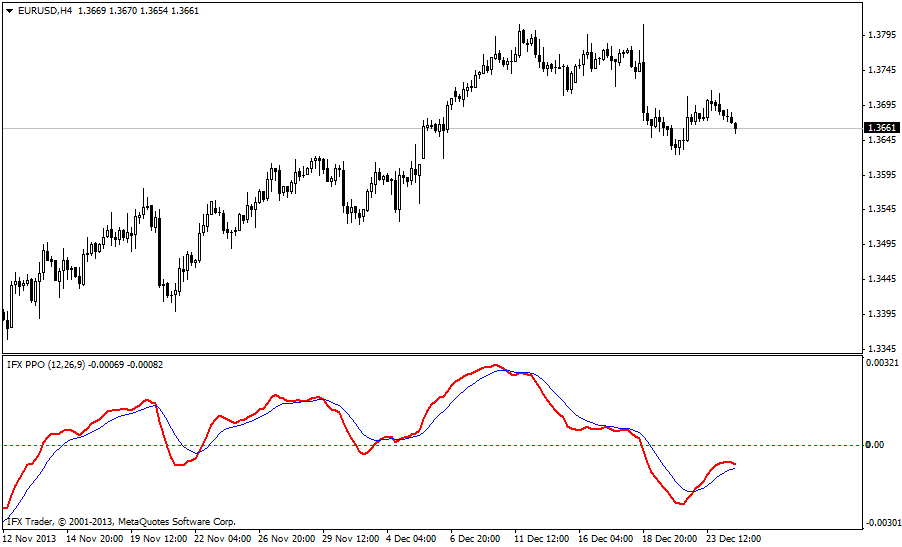# PPO Indicator

PPO is a technical momentum indicator measuring the difference between the 26-day and the 9-day exponential moving averages. The indicator is similar to the moving average convergence divergence (MACD) in terms of both practical use and efficiency.

#### Formula

PPO = (EMA(12) – EMA(26) / EMA(26)) x 100